# ConceptNet Numberbatch Word Vectors V17.06

Represent words as vectors

Released in 2016, these word representations were obtained by combining knowledge from the human-made ConceptNet graph and multiple pre-trained, distributional-based embeddings: GloVe, word2vec and the fastText algorithm trained on the Open Subtitles 2016 dataset. This net encodes more than 400,000 tokens as unique vectors, with all tokens outside the vocabulary encoded as the zero-vector. Underscores in the original model's tokens have been replaced with white spaces.

Number of layers: 1 | Parameter count: 125,158,500 | Trained size: 503 MB |

## Training Set Information

• ConceptNet, a knowledge graph that connects words and phrases of natural language with labeled edges. GloVe, word vectors pre-trained on various datasets. word2vec, word vectors pre-trained on the Google News dataset. Open Subtitles 2016, a collection of movie subtitles.

## Examples

### Resource retrieval

Get the pre-trained net:

 In:=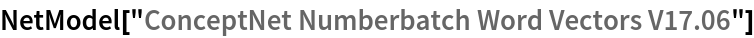Out=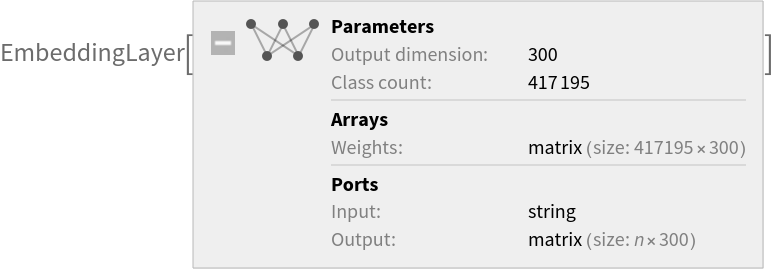### Basic usage

Use the net to obtain a list of word vectors:

 In:=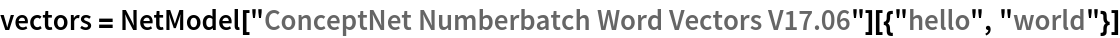Out=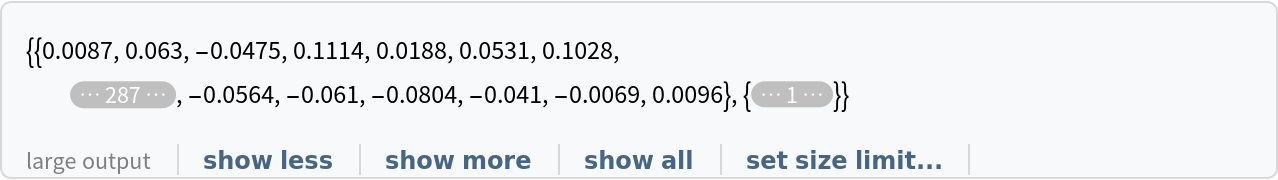Obtain the dimensions of the vectors:

 In:=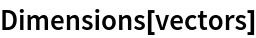Out=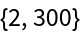Use the embedding layer inside a NetChain:

 In:=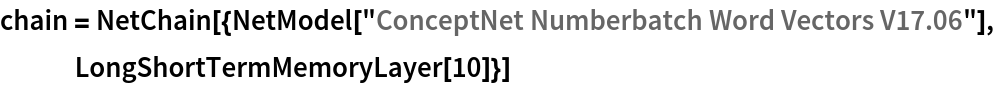Out=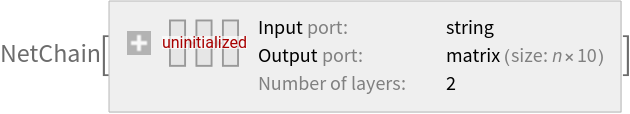### Feature visualization

Create two lists of related words:

 In:=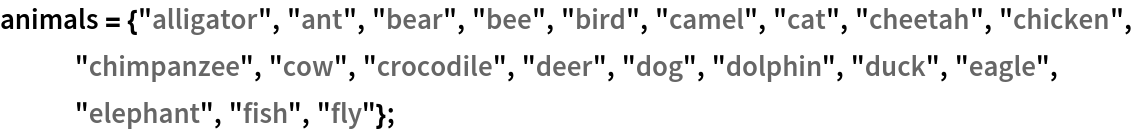In:=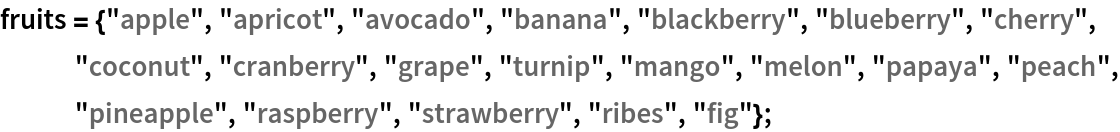Visualize relationships between the words using the net as a feature extractor:

 In:=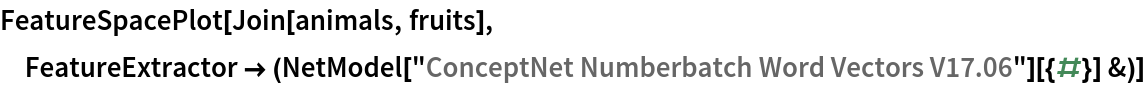Out=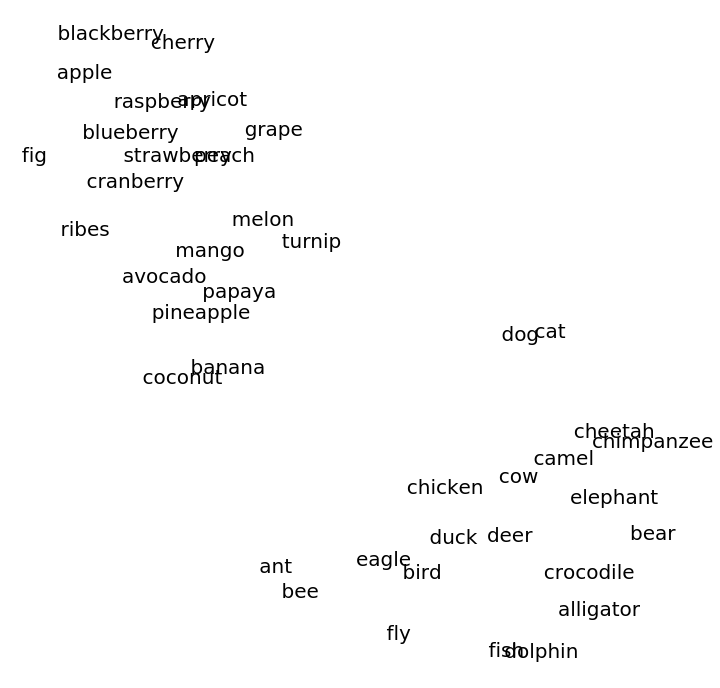### Word analogies

Get the pre-trained net:

 In:=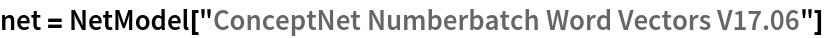Out=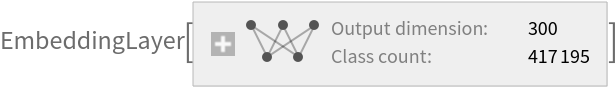Get a list of tokens:

 In:=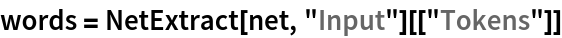Out=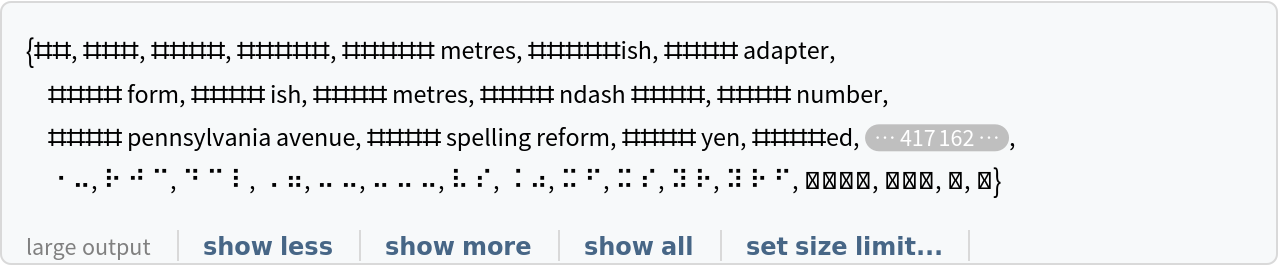Obtain the vectors:

 In:=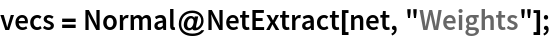Create an association whose keys are tokens and whose values are vectors:

 In:=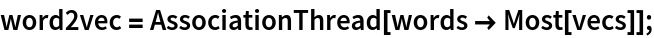Find the eight nearest tokens to "king":

 In:=Out=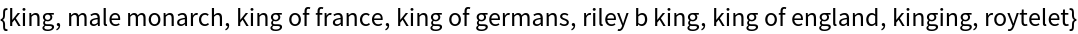France is to Paris as Germany is to:

 In:=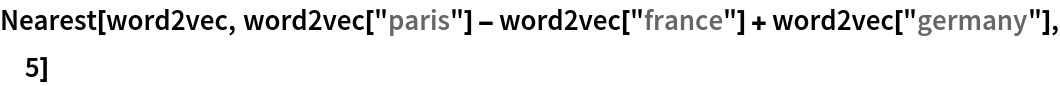Out=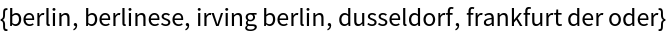### Net information

Inspect the number of parameters of all arrays in the net:

 In:=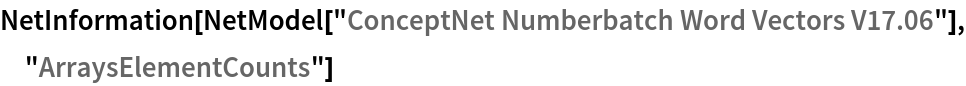Out=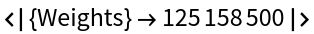Obtain the total number of parameters:

 In:=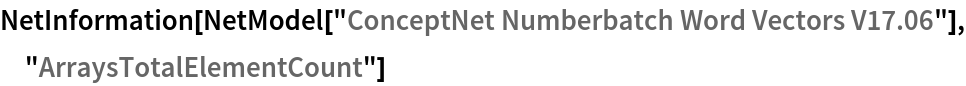Out=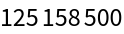Obtain the layer type counts:

 In:=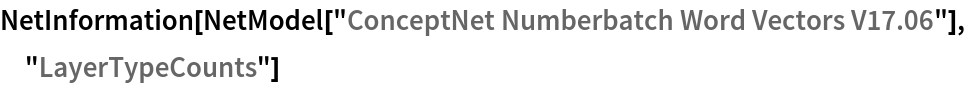Out=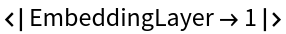### Export to MXNet

Export the net into a format that can be opened in MXNet:

 In:=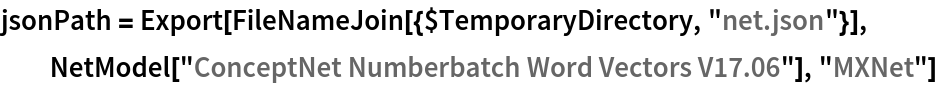Out=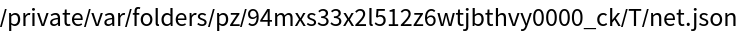Export also creates a net.params file containing parameters:

 In:=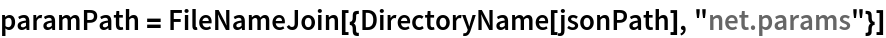Out=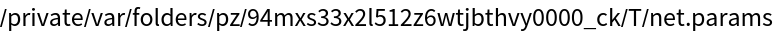Get the size of the parameter file:

 In:=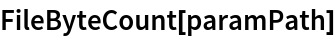Out=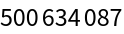The size is similar to the byte count of the resource object:

 In:=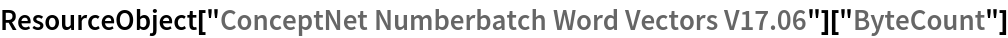Out=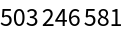Represent the MXNet net as a graph:

 In:=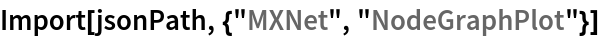Out=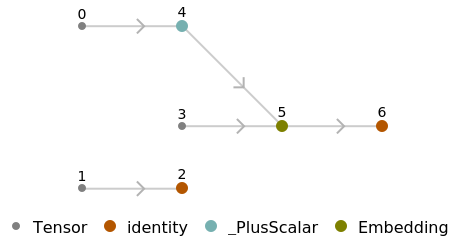## Requirements

Wolfram Language 11.3 (March 2018) or above